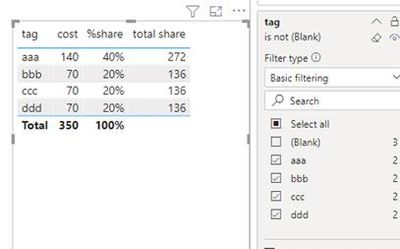cancel
Showing results for
Did you mean:Frequent Visitor

## measure to distribute specific row share among the other row values as per share percentage

I have a data something like below table, and the need is to distribute the blank tag cost among the other tag according to their related share of not blank value. Example..

 tag cost aaa 100 bbb 10 ccc 40 ddd 50 (blank) 80 bbb 60 ccc 30 ddd 20 aaa 40 (blank) 500 (blank) 100

Expected calculation

for aaa the share will be "140/(100+20+30+50+60+30+20+40)" = 40% of 680

for bbb the share will be  "70/(100+20+30+50+60+30+20+40)" = 20% of 680

for ccc the share will be "70/(100+20+30+50+60+30+20+40)" = 20% of 680

for ddd the share will be "70/(100+20+30+50+60+30+20+40)" = 20% of 680

expected solution

 tag cost %share total Share aaa 140 40% of 680 272 bbb 70 20% of 680 136 ccc 70 20% of 680 136 ddd 70 20% of 680 136

1 ACCEPTED SOLUTIONCommunity Support

Hi @anuj_a ,

According to your description, here’s my solution.

1. %share = DIVIDE(SUM([cost]),SUMX(FILTER(ALL('Table'),NOT(ISBLANK([tag]))),[cost]))
2.  total share = SUMX(FILTER(ALL('Table'),ISBLANK([tag])),[cost])*[%share]
3.  Unselect blankBest Regards,
Community Support Team _ kalyj

If this post helps, then please consider Accept it as the solution to help the other members find it more quickly.

3 REPLIES 3Frequent Visitor

thanks @v-yanjiang-msft  and @Greg_Deckler - your solutions worked with my POC data, however my prod data with more filters and columns has some problems with this solution.
May be I will share more relavant dummy data other time on separate question. for this query I am good. thanks for your assistance.Community Support

Hi @anuj_a ,

According to your description, here’s my solution.

1. %share = DIVIDE(SUM([cost]),SUMX(FILTER(ALL('Table'),NOT(ISBLANK([tag]))),[cost]))
2.  total share = SUMX(FILTER(ALL('Table'),ISBLANK([tag])),[cost])*[%share]
3.  Unselect blankBest Regards,
Community Support Team _ kalyj

If this post helps, then please consider Accept it as the solution to help the other members find it more quickly.Super User

@anuj_a Seems like:

``````Measure =
VAR __Total = SUMX(FILTER(ALL('Table'),[tag]<>BLANK()),[cost])
VAR __BlankTotal = SUMX(FILTER(ALL('Table'),[tag]=BLANK()),[cost])
VAR __TagTotal = SUM('Table'[cost])
VAR __Percent = DIVIDE(__TagTotal,__Total,0)
RETURN
__TagTotal + __Percent * __BlankTotal``````

Become an expert!: Enterprise DNA
External Tools: MSHGQM
Latest book!:
Mastering Power BI 2nd EditionDAX is easy, CALCULATE makes DAX hard...